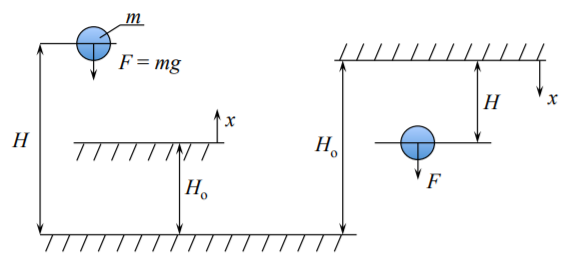# 7.1: The Concept of Potential Energy

$$\newcommand{\vecs}{\overset { \rightharpoonup} {\mathbf{#1}} }$$ $$\newcommand{\vecd}{\overset{-\!-\!\rightharpoonup}{\vphantom{a}\smash {#1}}}$$$$\newcommand{\id}{\mathrm{id}}$$ $$\newcommand{\Span}{\mathrm{span}}$$ $$\newcommand{\kernel}{\mathrm{null}\,}$$ $$\newcommand{\range}{\mathrm{range}\,}$$ $$\newcommand{\RealPart}{\mathrm{Re}}$$ $$\newcommand{\ImaginaryPart}{\mathrm{Im}}$$ $$\newcommand{\Argument}{\mathrm{Arg}}$$ $$\newcommand{\norm}{\| #1 \|}$$ $$\newcommand{\inner}{\langle #1, #2 \rangle}$$ $$\newcommand{\Span}{\mathrm{span}}$$ $$\newcommand{\id}{\mathrm{id}}$$ $$\newcommand{\Span}{\mathrm{span}}$$ $$\newcommand{\kernel}{\mathrm{null}\,}$$ $$\newcommand{\range}{\mathrm{range}\,}$$ $$\newcommand{\RealPart}{\mathrm{Re}}$$ $$\newcommand{\ImaginaryPart}{\mathrm{Im}}$$ $$\newcommand{\Argument}{\mathrm{Arg}}$$ $$\newcommand{\norm}{\| #1 \|}$$ $$\newcommand{\inner}{\langle #1, #2 \rangle}$$ $$\newcommand{\Span}{\mathrm{span}}$$$$\newcommand{\AA}{\unicode[.8,0]{x212B}}$$

From high school physics you must recall two equations

$E = \frac{1}{2} Mv^2 \quad \text{ kinematic energy}$

$W = mgH \quad \text{ potential energy}$

where $$H$$ is the hight of a mass $$m$$ from a certain reference level $$H_o$$, and $$g$$ stands for the earth acceleration. The reference level could be the center of the earth, the sea level or any surface from which $$H$$ is measured.Figure $$\PageIndex{1}$$: Gravitational potential energy.

We seldom measure $$H$$ from the center of earth. Therefore what we can easily measure is the change of the potential energy

$\Delta W = (mg)(H − H_o) \label{7.1.3}$

The energy is always positive. It can e zero but it cannot be negative. The gravity force $$F = mg$$ is directed towards the center of earth. Therefore there is a need for the negative sign in Equation \ref{7.1.3}). In the coordinate system of Figure ($$\PageIndex{1}$$), the gravity force is negative (opposite to the sense of coordinate $$x$$). The force $$F$$ is acting in the sense of $$x$$ but the difference $$H − H_o$$ is negative.

Extending the concept of the potential energy to the beam, the force is $$F = q dx$$ and the $$w = H − H_o$$ is the beam deflection.

$W \equiv + \int_{0}^{l} qw dx$

An analogous expression for plates is

$W \equiv + \int_{S} pw ds$Figure $$\PageIndex{2}$$: Potential energy of a beam element and the entire beam.

In the above definition $$W$$ is negative.

The concept of the energy stored elastically $$U$$ has been introduced earlier. For a 3-D body

$U = \int_{V} \frac{1}{2} \sigma_{ij}\epsilon_{ij} dv$

and for a beam

$U = \int_{0}^{l} \frac{1}{2} MK dx + \int_{0}^{l} \frac{1}{2} N \epsilon^{\circ} dx$

For plates, the bending and membrane energies are given by Equations (3.6.25), (3.6.26) and (3.6.41), (3.6.42).

The total potential energy $$\prod$$ is a new concept, and it is defined as the sum of the drain energy and potential energy

$\prod = U + (−W) = U − W$

Consider for a while that the material is rigid, for which $$U \equiv 0$$. Imagine a rigid ball being displaced by an infinitesimal amount on a flat $$(\theta = 0)$$ and inclined $$(\theta \neq 0)$$ surface, Figure ($$\PageIndex{3}$$).Figure $$\PageIndex{3}$$: Test if an infinitesimal displacement $$\delta u$$ causes the potential energy to change.

We have $$H = u \sin \theta$$ and $$\delta H = \delta u \sin \theta$$. The total potential energy and its change is

$\prod = −W = −F u \sin \theta$

$\delta \prod = −\delta W = −(F \sin \theta) \delta u$

On the flat surface, $$\theta = 0$$ and $$\delta \prod = 0$$, and the ball is in static (neutral) equilibrium. If $$\theta > 0$$, $$\delta \prod \neq 0$$ and the ball is not in static equilibrium. Note that if the d’Alambert inertia force in the direction of motion is added, the ball will still be in dynamic equilibrium. In this lecture, only static equilibrium is considered. We can now extend the above test for equilibrium and introduce the following principle:

The system is said to be in equilibrium, if an infinitesimal change of the argument $$a$$ of the total potential energy $$\prod = \prod(a)$$ does not change the total potential energy

$\delta \prod (a) = \frac{\partial \prod}{\partial a} \delta a = 0$

Because $$\delta a \neq 0$$ ($$\delta a = 0$$ is a trivial case in which no test for equilibrium is performed), the necessary and sufficient condition for stability is

$\frac{\partial \prod}{\partial a} = 0$

In case when the functional $$\prod$$ is a function of many (say $$N$$) variables $$\prod = \prod(a_i)$$, the increment

$\delta \prod = \frac{\partial \prod}{\partial a_i} \delta a_i, \quad i = 1, \dots, N$

The system is in equilibrium if the derivative of $$\prod$$ with respect to each variable at a time vanishes

$\frac{\partial \prod (a_i)}{\partial a_i} = 0, \quad i = 1, \dots, N$

The meaning of the argument(s) $$a_i$$, or independent variables will be explained next.

This page titled 7.1: The Concept of Potential Energy is shared under a CC BY-NC-SA 4.0 license and was authored, remixed, and/or curated by Tomasz Wierzbicki (MIT OpenCourseWare) via source content that was edited to the style and standards of the LibreTexts platform; a detailed edit history is available upon request.